Question

Determine the volume of 0.170 M NaOH solution required to neutralize each sample of hydrochloric acid. The neutralization reaction is:

NaOH(aq)+HCl(aq)→H2O(l)+NaCl(aq)

45 mL of a 0.050 M HCl solution

Express your answer to two significant figures and include the appropriate units.

165 mL of a 0.885 M HCl solution

Express your answer to three significant figures and include the appropriate units.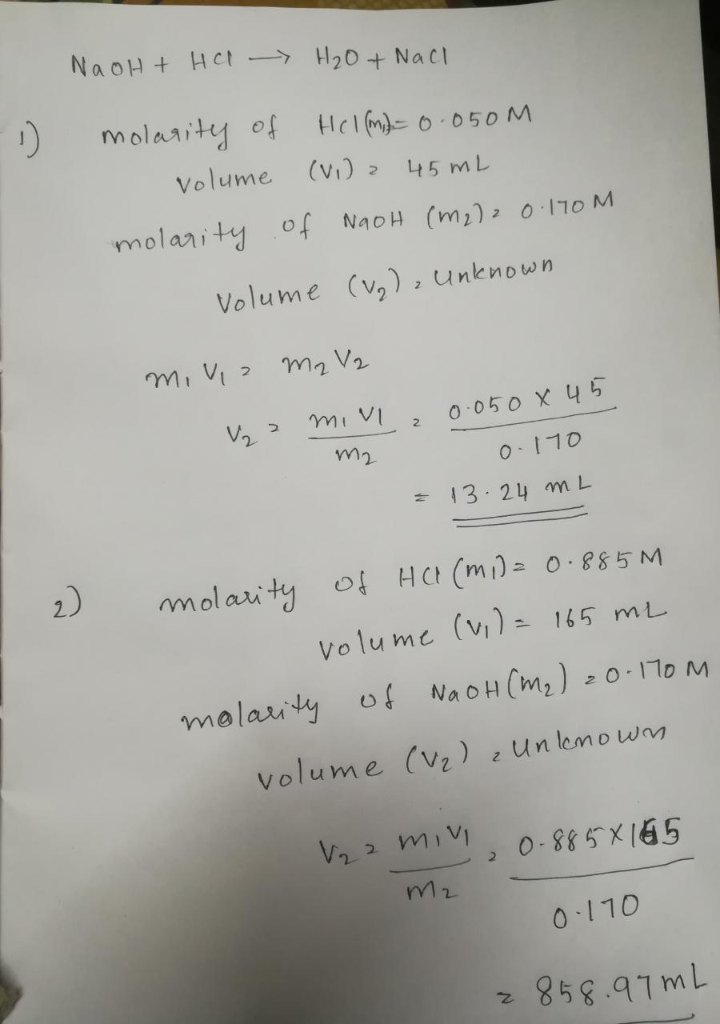#### Earn Coins

Coins can be redeemed for fabulous gifts.

Similar Homework Help Questions
• ### Determine the volume of 0.155 M NaOH solution required to neutralize each sample of hydrochloric acid.

Determine the volume of 0.155 M NaOH solution required to neutralize each sample of hydrochloric acid. The neutralization reaction is: NaOH(aq) + HCl(aq) → H2O(l) + NaCl(aq) Part A 30 mL of a 0.155 M HCl solution Part B 50 mL of a 0.070 M HCl solutionPart C195 mL of a 0.925 M HCl solution

• ### RA_CHEM151 <MC Chapter 13 Exercise 13.89 Determine the volume of 0.130 M N O solution required...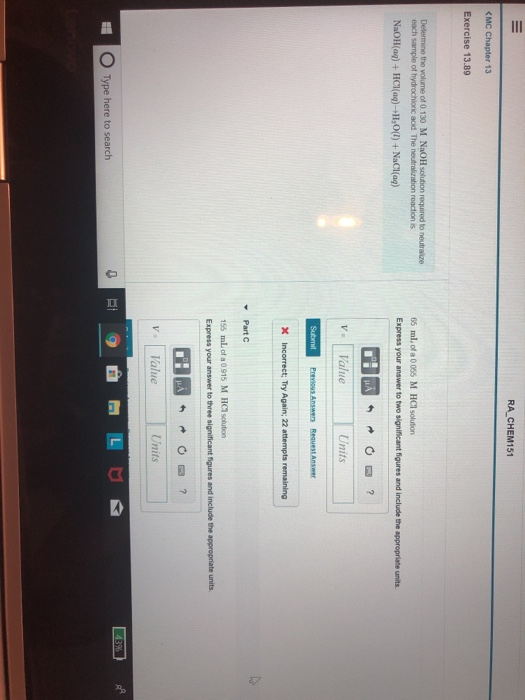RA_CHEM151 <MC Chapter 13 Exercise 13.89 Determine the volume of 0.130 M N O solution required to our each sample of hydrochon scd The neutr o n reaction is NaOH(aq) + HCl(aq) +,0(1) + NaCl(aq) 65 ml of a 0055 M HClotion Express your answer to two significant figures and include the appropriate units Value Units Pro Answers Revest Answer X Incorrect, Try Again 22 attempts remaining Part 155 m.ca 0915 M HCl solution Express your answer to three significant...

• ### Determine the volume of 0.150 M NaOH solution required to neutralize 40 mL of a 0.44...

Determine the volume of 0.150 M NaOH solution required to neutralize 40 mL of a 0.44 M HCl solution. The neutralization reaction is NaOH(aq) + HCl(aq) → H2O(l) + NaCl(aq)

• ### Determine the volume of 0.230 M KOH solution required to neutralize each sample of sulfuric acid. The neutralization reaction is:

Determine the volume of 0.230 M KOH solution required to neutralize each sample of sulfuric acid. The neutralization reaction is: H2SO4(aq)+2KOH(aq)→ K2SO4(aq)+2H2O(l)Part A25 mL of 0.230 M H2SO4. Express your answer using two significant figures.

• ### 9) 19.63 mL of 0.100 M NaOH is required to neutralize 2.00 mL of a solution...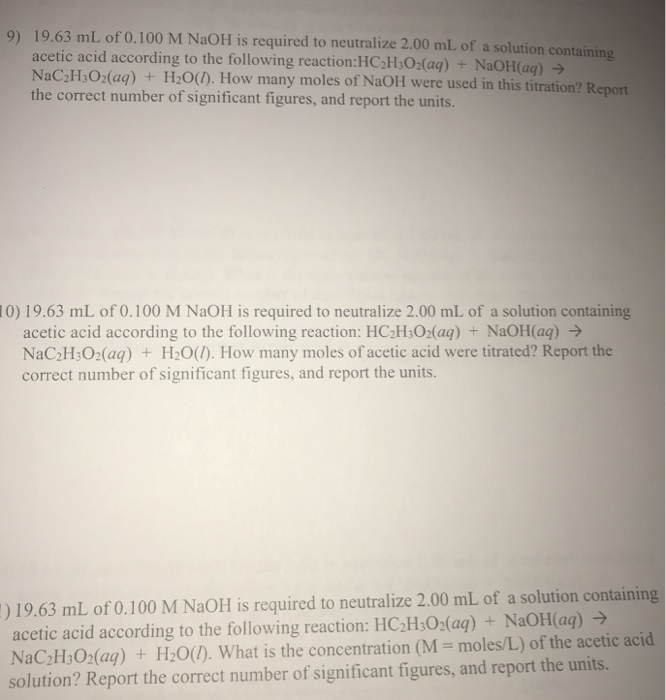9) 19.63 mL of 0.100 M NaOH is required to neutralize 2.00 mL of a solution containing acetic acid according to the following reaction:HC2H3O2(aq) + NaOH(aq) → NaC2H;O2(aq) + H2O(1). How many moles of NaOH were used in this titration? Report the correct number of significant figures, and report the units. 10) 19.63 mL of 0.100 M NaOH is required to neutralize 2.00 mL of a solution containing acetic acid according to the following reaction: HC2H302(aq) + NaOH(aq) → NaC2H:02(aq)...

• ### Please help I've struggled with this one for a bit now Review Const ✓ Correct Part...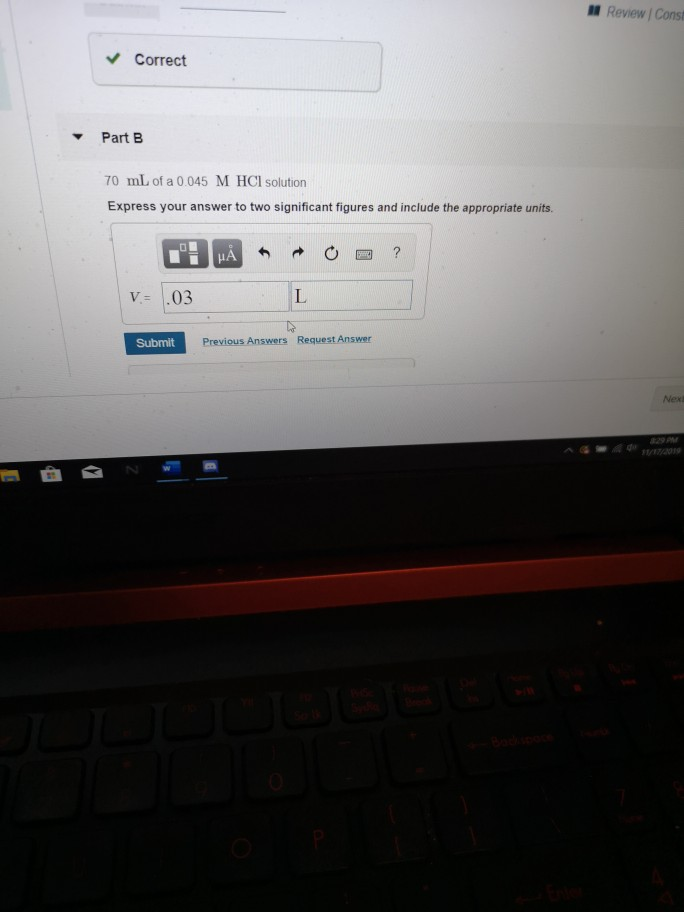Please help I've struggled with this one for a bit now Review Const ✓ Correct Part B 70 mL of a 0.045 M HCl solution Express your answer to two significant figures and include the appropriate units. I HÀ * * * E3 ? V.= .03 Submit Previous Answers Request Answer Nex Napel 13 HW Assignment <Chapter 13 HW Assignment Exercise 13.89 Review Constants Per Previous Answers Determine the volume of 0.120 M NaOH solution required to neutralize each sample...

• ### 2) 19.63 mL of 0.100 M NaOH is required to neutralize 2.00 mL of a solution containing acetic acid according to th...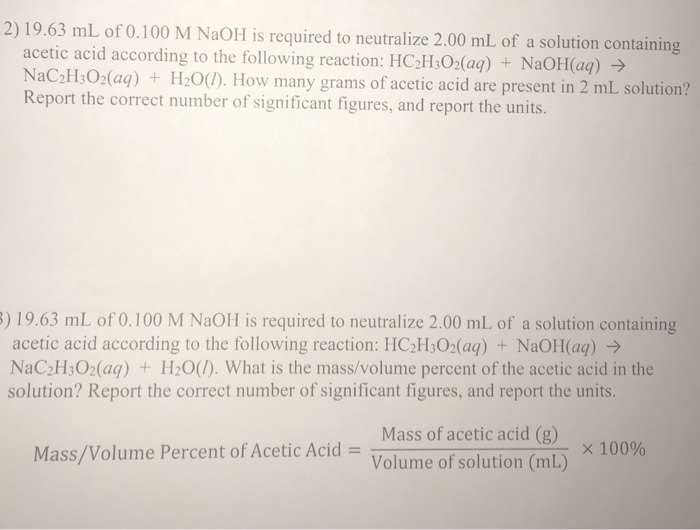2) 19.63 mL of 0.100 M NaOH is required to neutralize 2.00 mL of a solution containing acetic acid according to the following reaction: HC2H302(aq) + NaOH(aq) → NaC2H3O2(aq) + H2O(1). How many grams of acetic acid are present in 2 mL solution? Report the correct number of significant figures, and report the units. 3) 19.63 mL of 0.100 M NaOH is required to neutralize 2.00 mL of a solution containing acetic acid according to the following reaction: HC2H3O2(aq) +...

• ### If 32.12 mL of 3.77 M hydrochloric acid solution, HCl, are titrated with 51.5 mL of sodium hydroxide solution, NaOH...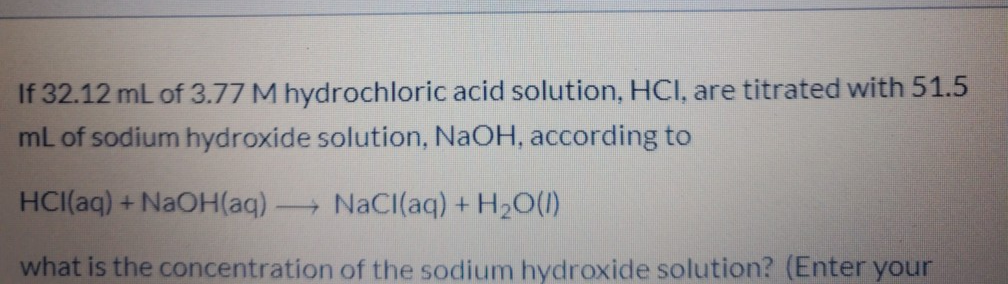If 32.12 mL of 3.77 M hydrochloric acid solution, HCl, are titrated with 51.5 mL of sodium hydroxide solution, NaOH, according to HCl(aq) + NaOH(aq) - NaCl(aq) + H2O(1) what is the concentration of the sodium hydroxide solution? (Enter your

• ### Determine the volume of 0.220 M KOH solution required to neutralize each sample of sulfuric acid....

Determine the volume of 0.220 M KOH solution required to neutralize each sample of sulfuric acid. The neutralization reaction is: H2SO4(aq)+2KOH(aq)→ K2SO4(aq)+2H2O(l) A.45 mL of 0.220 M H2SO4 B.195 mL of 0.120 M H2SO4 C.45 mL of 0.105 M H2SO4

• ### 1. What volume of 1.00 M HCl in liters is needed to react completely (with nothing...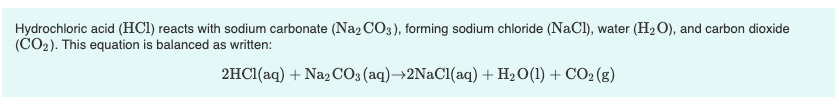1. What volume of 1.00 M HCl in liters is needed to react completely (with nothing left over) with 0.750 L of 0.200 M Na2CO3? Express your answer to three significant figures and include the appropriate units. 2. A 639-mL sample of unknown HCl solution reacts completely with Na2CO3 to form 15.1 g CO2. What was the concentration of the HCl solution? Express your answer to three significant figures and include the appropriate units. Hydrochloric acid (HCl) reacts with sodium...